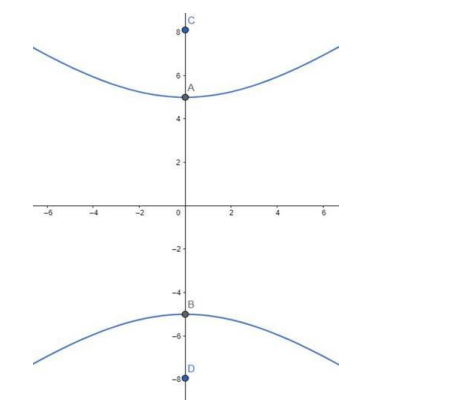# Find the equation of the hyperbola with vertices at`
Question:

Find the equation of the hyperbola with vertices at $(0, \pm 5)$ and foci at $(0, \pm 8)$.

Solution:

Given: Vertices at $(0, \pm 5)$ and foci at $(0, \pm 8)$

Need to find: The equation of the hyperbola.

Let, the equation of the parabola be:

$\frac{y^{2}}{a^{2}}-\frac{x^{2}}{b^{2}}=1$

Vertices of the parabola are at $(0, \pm 5)$

That means $\mathrm{a}=5$

The foci are given at $(0, \pm 8)$$A$ and $B$ are the vertices. $C$ and $D$ are the foci.

That means, ae $=8$, where $e$ is the eccentricity.

$\Rightarrow 5 \mathrm{e}=8[$ As $\mathrm{a}=5]$

$\Rightarrow \mathrm{e}=\frac{8}{5}$

We know that, $e=\sqrt{1+\frac{b^{2}}{a^{2}}}$

Therefore,

$\Rightarrow \sqrt{1+\frac{b^{2}}{a^{2}}}=\frac{8}{5}$

$\Rightarrow 1+\frac{b^{2}}{a^{2}}=\frac{64}{25}$ [Squaring both sides]

$\Rightarrow \frac{b^{2}}{a^{2}}=\frac{64}{25}-1=\frac{39}{25}$

$\Rightarrow b^{2}=a^{2} \frac{39}{25}$

$\Rightarrow \mathrm{b}^{2}=25 \times \frac{39}{25}=39[$ As $\mathrm{a}=5]$

So, the equation of the hyperbola is,

$\frac{y^{2}}{a^{2}}-\frac{x^{2}}{b^{2}}=1 \Rightarrow \frac{y^{2}}{25}-\frac{x^{2}}{39}=1$ [Answer]